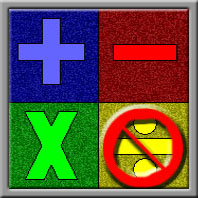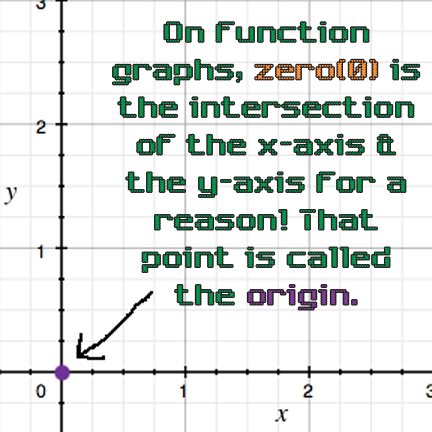# Zero: The Number of EmptinessThis character's name is Miss Zero Digit.

## This special number can be used in addition, subtraction, multiplication but not division! Multiplying by zero always gives you zero, but division by zero is undefined! (See Math Trick #48: "Multiplication Vs. Division By Zero" for more details)### Note: Since zero(0) is the number of additive identity, subtracting it from a number also gives you no change.

Have you noticed that whenever you have a quantity of objects, the quantity is always a natural number? Zero(0) is the number of emptiness because that's what it practically represents. When you say that you have no apples, you're saying that the number of apples that you have is zero(0). That means that you have no apples to give or lose. Furthermore, it also means that there is none to take from you. Yet, there are numbers that are less than zero(0)! However, according to the laws of physics, if the quantity is equal to zero(0), then it can't get any smaller because you can't get anything physical from emptiness. In fact, emptiness itself is a lack of something.

Zero(0) is not a natural number, which makes 1 the smallest natural number. With zero(0) included, you have the set of whole numbers. Despite the fact that some objects can be sliced or broken to give fractions, only positive integers count as natural numbers. Zero(0) is neither positive nor negative; it's the only neutral number.Referring to the image just above, functions are based on formulae, which are mathematical rules expressed in symbols. Most of these symbols are numbers, but some of them are variables that can have specific values. Numbers show up randomly in any formula because it’s part of their purpose. Numbers tell you how big something is or how many things you have or how great a quality or quantity is.

There are specific types of numbers that can’t be possible solutions for specific formulae because the formula would no longer make sense if those numbers were chosen values for the independent variables! For example, although 0 − 1 = −1, if you don’t have any oranges, (zero(0) oranges) then an orange (1 single orange)CAN’T be taken away from you! (How can you have negative one(-1) oranges? That doesn’t make any sense!)This character's name is Miss One First Digit.